## Friday, May 30, 2014

### HP Prime: Ways to use the INPUT command (Version 6030)

Note: This is for HP Prime Software Version 6030 (2014 3 31)

The INPUT Command

On the HP Prime, the INPUT command can include various types of input forms: numeric fields, choose boxes, and check boxes. You can also restrict which types of input are accepted. This blog entry will cover some of the basic forms of INPUT.

Basic Form

INPUT(var, title, label);

var = can be one or a list
title = optional string
label = optional string, number of labels are equal to number of var

The example programs presented (except for IN4) win use one variable.

Here is an example of the basic format. Keep in mind, the title and label strings are optional.

EXPORT IN1( )
BEGIN
// Basic
INPUT(X, "Enter X");
RETURN √(1+X^2);
END;

Specifying Types

INPUT( {var, [types allowed]}, title, label);

Type numbers are surrounded in square brackets.

Common Types:
-1 All
0 real numbers
1 integers
2 strings
3 complex numbers
4 matrices
6 lists
8 functions
9 numbers with units
14.? CAS objects

IN2 and IN3 are programs that ask for a specific type. IN2 asks for a matrix (type 4) and IN3 asks for a list (type 6).

EXPORT IN2( )
BEGIN
INPUT({{M0,}}, "Matrix");
RETURN M0*TRN(M0);
END;

EXPORT IN3( )
BEGIN
INPUT({{L0, }}, "List");
PRINT( );
PRINT("Σx ="+STRING(ΣLIST(L0)));
PRINT("mean = "+STRING(approx(mean(L0))));
PRINT("std dev ="+STRING(approx(steddevp(L0))));
END;

Checkboxes

INPUT({{var, n}}, title, label);

n groups the current and next n-1 checkboxes. If you want a checkbox next to each variable, let n = 1. A variable that is checked has a value of 1. Otherwise, the value is 0. Each variable and it's corresponding n are surrounded in curly brackets.

EXPORT IN4( )
BEGIN
// Checkboxes
INPUT( {{A,1}, {B,1}, {C,1}}, "Turn On Variables");
PRINT( );
PRINT("Number of Checks: "+STRING(A+B+C));
END;

If you want to use more than one input form, I advise to keep INPUT types separate.

EXPORT IN5( )
BEGIN
// Checkbox Modes
INPUT( {{M,1}}, "Check for Degrees");
HAngle:=M;
// Input Data
INPUT(A, "Angle");
PRINT( );
PRINT("SIN(2A)² =");
PRINT(SIN(2*A)²);
END;

Choose Boxes

This INPUT form will create a choose box (drop down list).

INPUT( {{ var, {choice0, choice1, choice2...} }}, title, label)

IF var == 0 THEN choice0 instructions...(END;)
ELSE (if there are only two choices) or
IF var ==1 THEN choice1 instructions... END; and so on...

EXPORT IN6( )
BEGIN
INPUT( {{C, {"SI","US"} }}, "Units of g");
IF C==0 THEN
RETURN "g = 9.80665 m/s^2"; KILL;
ELSE
RETURN "g = 32.17404 ft/s^2"; END;
END;

That is some of the ways we can use the INPUT command on the HP Prime. To check out all of the ways INPUT command, including putting the prompts in specific places, check out the help screen for INPUT.

Eddie

This blog is property of Edward Shore. 2014

## Wednesday, May 28, 2014

### Retro-Review: Casio Algebra FX 2.0 Plus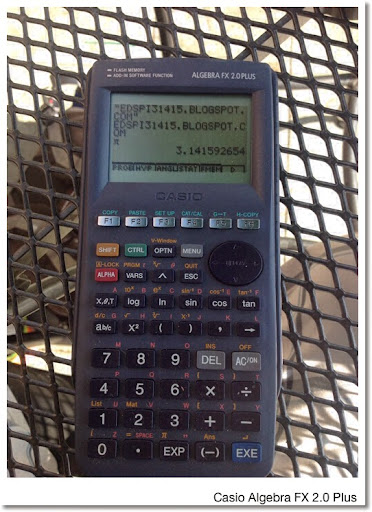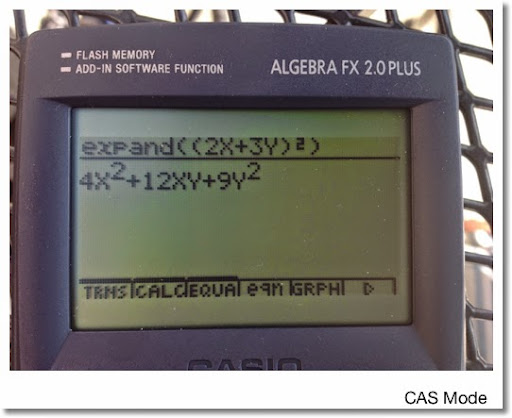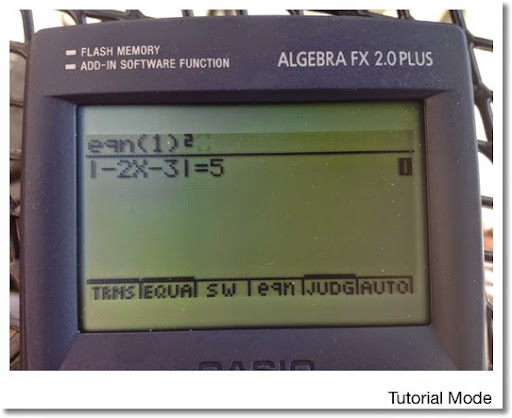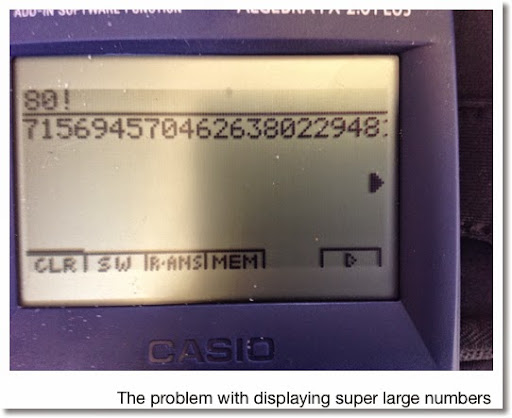Retro-Review: Casio Algebra FX 2.0 Plus

Production Period: 1999 - 2001 (thank you www.rskey.org)
Memory: 146,000 bytes with 768,000 bytes flash

On the surface, the Casio Algebra FX 2.0 Plus looks like a typical modern Casio graphing calculator: the MENU key displays an iconic menu, the top row is a set of six soft keys, the layout of the keys are similar, and its programming language is the same as its fellow graphic calculators.

I am just going to go over what separates the Algebra FX 2.0 Plus from the other Casio calculators. We can assume it can handle what ever the fx-9860g series (including the Prizm, sans color) does.

What separates the Algebra FX 2.0 from the other Casio graphing calcualtors? First, complex numbers are not only allowed as arguments in transcendental functions (logarithmic and trigonometric functions). Complex numbers are accepted as coefficient in the polynomial and simultaneous equation solvers. Second, instead of the usual 6, the polynomial and simultaneous equations solvers work orders up to 30. The big difference, is that calculator has CAS (computer algebraic system) capabilities.

Being Casio, the CAS functionality is accessed in a separate mode. Make that three separate modes.

With each CAS mode allowing equations, algebraic expressions, integers, and fractions to be expanded, factored, simplified, and approximated; the mode dictated which other options were available:

CAS Mode: This had the full suite of CAS functions on the Algebra FX 2.0 Plus: derivative, integral, sums, the ability to work with ∞, extracting parts of an expression, calculating arc length, tangent lines, GCD, and LCM. (I think I got most of it, it not all.)

Algebra Mode: This is a limited CAS mode, probably aimed at younger students. Only x!, |x|, denominator and numerator extraction, LCM, and GCD are available. Not sure what the Algebra mode exclusive arrange and replace commands do.

Tutorial Mode: Choose from a library of 45 equation types and use the CAS commands to solve them. What commands are available is dependent on the type of equation being solved.

Something I thought was strange with the CAS mode was that it could handle numbers bigger than 10^99. Try to get the approximation of that number, you get an overflow error. And really large numbers have digits cut off in the display so reading them presented a challenge.

For example: 80! (80! ≈ 7.1569 × 10^118)

Display: 7156945704626380229481(?) 6945704626380229481153(?)...

Trying approx(80!) returns an overflow error.

A impressive mode was the Differential Equation mode, where you can get a slope field plot of various types of equations including:
* 1st Order Linear
* 1st Order Bernoulli
* 1st Order Exact
* 2nd Order Linear and higher
* 1st Order Linear Systems

I bought my Algebra FX 2.0 I think more than a decade ago on clearance. I think this calculator was underrated due to its short production life. Was the Algebra FX 2.0 a predecessor to the ClassPad Series? Seems like it.

That is my retro-view. Hope you liked it. Talk to you next time!

Eddie

This blog is property of Edward Shore. 2014

## Sunday, May 25, 2014

### PCalc and HP 50g: Internal Pressure in a Vessel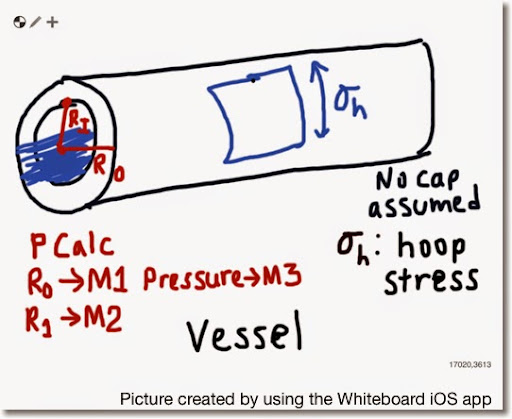PCalc and HP 50g: Internal Pressure in a Vessel

I = inner radius of the vessel
O = outer radius of the vessel
P = pressure of the water flow
σh = hoop stress

σh = P * (O^2 + I^2)/(O^2 - I^2)

Source: Goswami, Indramil Ph.D. P.E. "All In One Civil Engineering PE Breadth and Depth Exam Guide" 2nd Edition. McGraw Hill: 2012

PCalc: Pressure In A Vessel

Store the following:
I → M1
O → M2
P → M3

Decimal Mode
Set R1 To M1
R1 To Power of 2
Set R2 To M2
R2 To Power of 2
Set X To R1
Set R0 To R1
Subtract R2 From R0
Divide X By R0
Multiply X By M3

HP 50g: VESSEL

"Pressure" PROMPT
→ I O P
<< O SQ I SQ DUP2
+ UNROT - / P * →NUM
"σh" →TAG >> >>

Example:
O = 3/8 in = .375 in
I = 3/32 in = .09375 in
P = 10 lb/(s*in^2)

Result:
σh ≈ 11.3333 lb/(s*in^2)

- Eddie -

### PCalc and HP 50g: Horizontal Pipe Discharge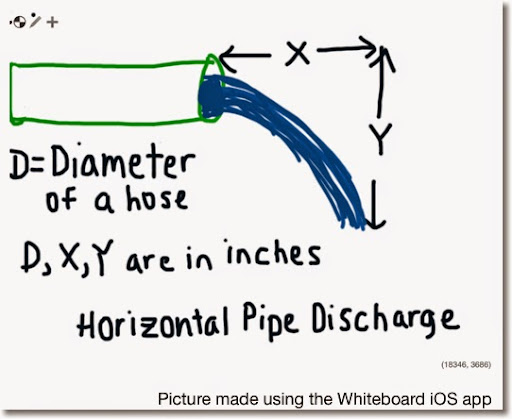PCalc and HP 50g: Horizontal Pipe Discharge

Variables:
X, Y, and D (diameter of the pipe) are in inches. Flow (Q) is calculated in GPM (gallons per minute).

Q ≈ .25974 * π * D^2/4 * X * √( g /(2 * Y))

Where
g = 9.80665 m/s^2 ≈ 386.08858 in/s^2
1 in^3/s ≈ .25974 gal/min

Source:

Gary P. Markey. Lecture 14: Flow Measurement in Pipes. BIE 5300/6300 Lectures
Utah State University - Open Courseware

http://ocw.usu.edu/Biological_and_Irrigation_Engineering/Irrigation___Conveyance_Control_Systems/L14_FlowMeasurementInPipes.pdf

PCalc: Horiz. Pipe Discharge (GPM)

Store the following values before proceeding:
D in M1
X in M2
Y in M3

Decimal Mode
Set X To M1
X To Power of 2
Multiply X By M2
Multiply X By Pi
Divide X By 4
Set R0 To 386.08858
Divide R0 By 2
Divide R0 By M3
R0 To Power of 0.5
Multiply X By R0
Multiply X By .25974

HP 50g: HPD

SQ: x^2

<< "Hose Diameter (in)" PROMPT
"X (in)" PROMPT
"Y (in)" PROMPT
→ D X Y
<< π D SQ * X * 4 / →NUM
386.08858 2 Y * / √ *
.25974 * "Flow (GPM)" →TAG >> >>

Example:
D = 2.5 in
X = 8 in
Y = 4 in

Result:
Flow ≈ 70.85936 Gallons per Minute

- Eddie -

### PCalc and HP 50g: Mohr's CirclePCalc and HP 50g: Mohr's Circle

Given shear stresses in the x and y directions (σx and σy) and normal stress (τ), find the radius, center, and viewing angle of Mohr's Circle.

I take τ to represent both τxy and τyx (both are assumed to be equal).

R = √( (σx - σy)/2)^2 - τ^2 )

Center (S, 0):
S = (σx + σy)/2

Viewing Angle:
θ = atan( 2τ /(σx - σy)) / 2

Sources:

HP 35S Program by Jason Charalambides, Avant Garde Engineering. HP 35S program written in 2012.

http://www.avant-garde-engineering.com/HP35s_Programs/Mohr's%20Circle.pdf

Wikipedia Article on Mohr's Circle, retrieved 5/23/2014:

http://en.wikipedia.org/wiki/Mohr's_circle

PCalc:

Before running, store the following values:
σx into M1
σy into M2
τ into M3

Decimal Mode
Set X To M1
Subtract M2 From X
Divide X By 2
X To Power of 2
Set R0 To M3
R0 To Power of 2
X To Power of 0.5

Program: Mohr's Circle - Center (X,0)
Decimal Mode
Set X To M1
Divide X By 2

Program: Mohr's Circle - View Angle
Decimal Mode
Set X To M3
Multiply X By 2
Set R0 To M1
Subtract M2 From M0
Divide X By R0
Inverse Tangent X
Divide X By 2

HP 50g: MOHR

σ: ALPHA, Right-Shift, S
τ: ALPHA, Right-Shift, U
SQ: x^2

<< "Shear Stress σx" PROMPT
"Shear Stress σy" PROMPT
"Normal Stress τ" PROMPT
→ X Y T
<< X Y - 2 / SQ T SQ + √
X Y + 2 / (0,0) +
"Center" →TAG
T 2 * X Y - / ATAN 2 /
"View Angle" →TAG >> >>

Example:
σx = 100
σy = -220
τ = 80

Results:
R ≈ 178.88544
S = -60 (center (-60,0))
θ ≈ 13.28253° ≈ .23183 radians

- Eddie -

## Friday, May 23, 2014

### PCalc and HP 50g: Newton's Law of Gravity Solver

PCalc Program: Newton's Law of Gravity (U.S. units)
5/20/2014

F = (G * m1 * m2) / r^2

A solver program for PCalc. Turn Multiple Memories on. Use the Skip If Equal command to simulate a menu.

M0 = F (force: ft^3/(lb s^2))
M1 = m1 (mass of object 1 in pounds)
M2 = m2 (mass of object 2 in pounds)
M3 = r (distance between center of masses in feet)

Enter 0 (zero) for any variable you want to solve for.

Program:
Decimal Mode
Set X To 1.0690466104e-9
Skip 3 If M0 = 0
Skip 7 If M1 = 0
Skip 13 If M2 = 0
Skip 19 If M3 = 0
Multiply X By M1
Multiply X By M2
Power M3 To -2
Multiply X By M3
Stop
Invert X
Multiply X By M0
Invert M2
Multiply X By M2
Power M3 To 2
Multiply X By M3
Stop
Invert X
Multiply X By M0
Invert M1
Multiply X By M1
Power M3 To 2
Multiply X By M3
Stop
Multiply X By M1
Multiply X By M2
Invert M0
Multiply X By M0
Power X To 0.5
Stop

Example:
M0 = 0 (solve for F)
M1 = 4.384e30
M2 = 1.317e25
M3 = 49079712000 = 92954000 × 5280

Solve: X = 2.562411e25 (F)

HP 50g: Newton's Law of Gravity Solver

F = force (ft^3)/(lb s^2)
M1 = mass of object 1 (lb)
M2 = mass of object 2 (lb)
R = distance between objects (ft)

Program GRAVITY
<< {'F' 'M1' 'M2' 'R'} PURGE F M1 M2 1.0690466104E-9
* * R SQ / = STEQ 30 MENU >>

This blog is property of Edward Shore. 2014

### PCalc and HP 50g: Period of Orbit Around the Sun

To determine the period of a satellite's orbit around the sun (mainly planet), Newton's version of Kepler's Third Law is used.

T = 2 π √(a^3 /(G * (MS + m)) )

Source: Astronomy 801: Planets, Stars, and the Universe - Penn State University
https://www.e-education.psu.edu/astro801/

PCalc Program: Period Around the Sun
5/19/2014

G = 1.0690441604e-9 ft^3/(s^2 lb)
Mass of the Sun = MS = 4.384e30 lbs

T = 2 π √(a^3 /(G * (MS + m)) )

Input:
Y: mass in pounds
X: distance in miles

Output:
X: period in years

Program:
Decimal Mode
Multiply X By 5280
X To Power of 3
Multiply Y By 1.0690441604e-9
Divide X By Y
X of Power of 0.5
Multiply X By 2
Multiply X By Pi
Divide X By 31556925.9747

Example:
Input:
Y: 1.3170e25 pounds (Mass of the Eath)
X: 9295400 miles (average semi-major axis between Earth and Sun)

Output:
X: 1.0000102186
It takes about 1.00001 years for Earth to orbit the Sun

HP 50g: Period Around the Sun:

Input:
2: mass of orbiting object in pounds
1: distance from sun in miles

Output:
1: period of orbit in years

Program PERSUN
<< 5280 * 3 ^ SWAP 4.384E30 +
1.0690441604E-9 * / √ 2 * π *
→NUM 31556925.9747 / >>

This blog is property of Edward Shore. 2014

### PCalc and HP50g: Distance of a Star by Parallax

Source: Astronomy 801: Planets, Stars, and the Universe - Penn State University
https://www.e-education.psu.edu/astro801/

PCalc Program: Distance of a Star by Parallax (Degrees Mode)
5/19/2014

D = approximate distance from Earth to the star
P = angle in arc seconds (1/3600 of the degree)

D = 1/ (tan(P/3600) * 63239.7263452)

1 light year = 63239.7263452 AU

(AU: distance from Earth to the Sun)

Input:
X: angle in arc seconds

Output:
X: distance in light year

Program:
Decimal Mode
Degrees Mode
Divide X By 3600
Tangent X
Multiply X By 63239.7263452
Invert X

Example:
Input:
X: 0.54 arc second

Output:
X: 6.0400619278 light years

HP 50g: Distance of a Star by Parallax

The commands PUSH and POP are used to preserve and recall settings. PUSH stores the settings while POP recalls stored settings. This allows the program to make necessary changes to program modes during execution with permanently affecting the settings for the user.

Input:
1: angle in arc seconds

Output:
1: distance to star in light years

Program PARALLAX
<< PUSH DEG 3600 / TAN
63239.7263452 * INV POP >>

This blog is property of Edward Shore. 2014

### PCalc and HP 50G: Escape Velocity

The next four posts will have programs for the PCalc iOS app and the HP 50g calculator. English/U.S. Units (feet/miles, pounds, seconds) will be used instead of SI units (meters/kilometers, kilograms, seconds).

The Universal Gravitational Constant is:

SI Units:
G = 6.67384 x 10^-11 m^3/(s^2 kg)

English Units:
G = 1.06904661604 x 10^-9 ft^3/(s^2 lb)

PCalc Program: Escape Velocity (in mi/sec)
5/19/2014

G = 1.06904661604 x 10^-9 ft^3/(s^2 lb)

Input:
Y: mass (in pounds)
X: distance or radius (in miles)

Output:
Y: mass (in pounds)
X: escape velocity (mi/sec)

Program:
Decimal Mode
Multiply X By 5280
Invert X
Multiply X By Y
Multiply X By 2
Multiply X By 1.0690441604e-9
X To Power of 0.5
Divide X By 5280

Example:
Y: 1.3170e25 lbs (Mass of Earth)
X: 3,959 mi (radius of Earth)

Output:
Y: 1.3170e25
X: 6.9512

Escape Velocity: 6.9512 mi/sec

HP 50g: Escape Velocity:

Input:
2: mass in pounds
1: distance in miles

Output:
1: escape velocity in mi/sec

Program ESCVEL
<< 5280 * INV * 2 * 1.0690441604E-9 * √ 5280 / >>

This blog is property of Edward Shore. 2014

## Friday, May 16, 2014

### fx-5800p Programs (can apply to Casio graphing calculators also, e.g. fx-9860g, Prizm)

This is a collection of programs I wrote on the Casio fx-5800p. These programs should also work on any Casio Graphing calculator (fx-9860g, fx-9750g, Prizm) since the programming language between Casio calculators remains largely the same.

Now if I can find my fx-5800p that I misplaced last night... *sigh*. Thank goodness for notes!

Notes for the fx-5800p programs:

There are no SIGN or MOD functions. Here are the work arounds I used (see SUN):

SIGN(x):
...
X > 0 ⇒ 1 → X
-X > 0 ⇒ -1 → X
...

n MOD d:
...
N - D Intg( N ÷ D ) → result variable
....

1. Rotation of (x, y) (ROTATEXY)
2. Law of Cosines (COSINES)
3. Pendulum: Period and Average Velocity (PENDULUM)
4. Arc Length of a Parabola (QUADLENGTH)
5. Position of the Sun (SUN)

1. fx-5800p: ROTATEXY

Rotates the coordinate (X, Y). The direction of rotation follows the conventional direction (counterclockwise). The variable A represents the angle (θ).

Program:
"X"? → X
"Y"? → Y
"ANGLE"? → A
[ [ X, Y ] ] × [ [ cos(A), sin(A) ] , [ -sin(A), cos(A) ] ]

2. fx-5800p: COSINES

Sides: D, E, F
Corresponding Angles: A, B, C

Program:
Lbl 0
Cls
"KNOWN:"
"1. D,E,F"
"2. A,E,F"
?→I
I = 1 ⇒ Goto 1
I = 2 ⇒ Goto 2
Goto 0
Lbl 1
"D"? → D : "E"? → E : "F"? → F
"A" : cos ⁻¹ (( E ² + F ² - D ² ) ÷ ( 2EF )) → A ◢
"B": cos ⁻¹ (( D ² + F ² - E ² ) ÷ ( 2DF )) → B ◢
"C" : 180° - A - B
Stop
Lbl 2
"A"? → A : "E"? → E : "F"? → F :
"D": √ (E ² + F ² - 2 E F cos A ) → D ◢
"B" : cos ⁻¹ ( ( D ² + F ² - E ² ) ÷ (2DF)) → B ◢
"C": 180° - A - B

3. fx-5800p: PENDULUM

Variables:
D = length of the step or bar holding the pendulum
L = length of the rod or string that is swinging
R = large radius of the circular ring

At the units, enter 0 for US units (set g = 32.174 ft/s^2), anything else for SI units (g = 9.80665 m/s^2).

Calculated:
T = period of the pendulum (the amount of time it takes for the pendulum from one end to the other)
V = average velocity of the pendulum (once in its in full swing)

Program:
Cls
"=0 U.S."
"≠0 SI"
? → G
If G = 0
Then 32.174 → G
Else g → G IfEnd // g from the constant menu (9.80665)
Lbl 0
Cls
"1. ROD 2. STRING"
"3. RING"
?→ I
I = 1 ⇒ Goto 1
I = 2 ⇒ Goto 2
I = 3 ⇒ Goto 3
Goto 0
Lbl 1
"D"? → D : "L"? → L
2 π √( L ÷ 3G ) → T : Goto 4
Lbl 2
"D"? → D : "L"? → L
2 π √(L ÷ G) → T : Goto 4
Lbl 3
"D"? → D : "L"? → L : "R"? → R
2 π √( R ² ÷ GL → T : Goto 4
Lbl 4
"T =" : T ◢ "V =": D ÷ T → V

-----

Test Examples:

Rod: D = 1 m, L = 1.6 m. Results: T = 14.36943096 sec, V = .0695921782 m/s

String: D = 2 m, L = 1.75 m. Results: T = 2.65423008 sec, V = .07535141995 m/s

Circular Ring: D = 2 ft, L = 2 ft, r = 1.2 ft
Results: T = 1.879851674 sec, V = 1.063913727 m/s

Find the length of a parabola given height and width and a corresponding equation:

f(x) = Ax^2 + Bx

Where
A = -4h/l^2
B = 4h/l

Program:
Cls
"LENGTH"? → L
"HEIGHT"? → H
Cls
"COEF OF X ²"
-4 H ÷ L ² ◢
"COEF OF X"
4 H ÷ L ◢
"ARC LENGTH"
∫ ( √ ( 1 + ( -8 H X ÷ L ² + 4 H ÷ L ) ) , 0, L)

Test Data:
L: 16.4, H: 8.2
X^2 coefficient: -.1219512195
X coefficient: 2

5. fx-5800P: SUN

Source for the formulas: http://aa.usno.navy.mil/faq/docs/SunApprox.php

Gives the RA (right ascension) and δ (declination) of the sun at any date. U is the universal time, the time it would be at Greenwich Village (Int'l Date Line).

For the Pacific Time Zone:
Standard Time: PST + 8 hours = UT
Daylight Savings Time: PDT + 7 hours = UT

Program:
"MONTH"? → M
"DAY?" → D
"YEAR"? → Y
"UNIV. TIME"? → U
Deg
100 Y + M - 190002.5 → X
X > 0 ⇒ 1 → X
-X > 0 ⇒ -1 → X
367 Y - Intg( 7 ( Y + Intg( ( M + 9 ) ÷ 12 ) ) ÷ 4 )
+ Intg( 275 M ÷ 9 ) + D + 1721013.5 + U ÷ 24
- .5 X + .5 → D
D - 2451545 → D
357.529 + .98560028 D → G
G - 360 Intg( G ÷ 360 ) → G
280.459 + .98564736 D → Q
Q - 360 Intg( Q ÷ 360 ) → Q
Q + 1.915 sin( G ) + .02 sin( 2G ) → L
L - 360 Intg( L ÷ 360 ) → L
1.00014 - .01671 cos( G ) - .00014 cos( 2 G ) → R
23.439 - .00000036 D → E
tan ⁻¹ (cos(E) tan(L)) → A
If L ≥ 90 And L < 270
Then A + 180 → A
IfEnd
If L ≥ 270 And L < 360
Then A + 360 → A
IfEnd
A ÷ 15 → A
sin ⁻¹ ( sin(E) sin(L) ) → C
"APPROX:"
"RA (HRS)": A ◢
"DEC (DEG)": C

Test Data:
M: 5
D: 14
Y: 2014
U: 19

RA: 3.43 hours
DEC: 18.746°

Eddie

This blog is property of Edward Shore. 2014

## Sunday, May 11, 2014

### Scientific Keyboard Challenge, Revisited

On this post:

http://edspi31415.blogspot.com/2014/05/scientific-calculator-keyboard-challenge.html,

I have issued a creativity challenge: design a scientific calculator using only 25 keys.  No restrictions.  I came up with a couple ideas.

Before I present mine, I want to show a great landscape design by Picky Bart.  Thanks for posting PickyBart!:

http://pickyb.blogspot.co.uk/2014/05/proposed-scientific-rpn-hp-quickcalc.html

---------------------

The first one is an RPN keystroke programmable calculator:

The next one is a graphing calculator. (or at least an attempt at one).  Since only 25 keys are allowed, this prevents the use of an ALPHA key.  So, soft keyboards via dialogue had to be implemented instead.Modes: RPN/Linear/Math IO DEG/RAD/GRAD FUNC/POLAR/PARAM/SEQN a+bi, r< , >, >=; <, <=, and, or IFTE:  If, Then, Else, EndIf Loop:  For, Step, Next; Repeat, Until, While, Wend Ctrl:  Pause, Stop, Error, Subr String:  length, sub, left, right; >expr, >string, position, augment Math: Frac:  PDQ, a b/c, exact, approx Hyp:  (hyperbolic) Prob: x!, nCr, nPr, gamma; rand, randint, randnorm, dice Cmplx: abs, arg, real, imag; >Polar, >Rect Parts: IP, FP, round Calc:  derivative, integral, S, P Constants: (24) Conversions: (12 pairs) Base: Mark: b, o, h, d HEX: A, B, C, D; E, F Logic: and, or, not, xor; xnor, shift right, shift left, 2's Utility: RPN: swap, roll down, roll up, drop; over, pick, view, dupn Solver: New, Edit, Delete, Solve ALPHA: - enter message and press enter CAPS (Keyboard), lower (keyboard), Symb (! @ # \$ % ^ & * ( ) { } and math symbols), Greek  I may have forgot something but I think I got all the basics covered.
This post is property of Edward Shore  - 2014

﻿

### HP 15C: Error Function and Lower Tail Normal Cumulative Function

HP 15C:   Error Function and Lower Tail Normal Cumulative Function Formulas Used  Error Function  erf(x) = 2 ÷ √π * ∫( e^(-t^2) dt, t = 0 t...